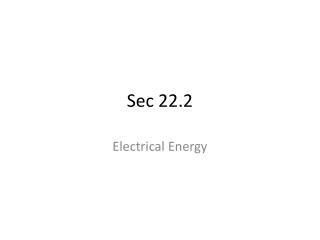DownloadDownload PresentationSec 22.2

# Sec 22.2

Download Presentation## Sec 22.2

- - - - - - - - - - - - - - - - - - - - - - - - - - - E N D - - - - - - - - - - - - - - - - - - - - - - - - - - -
##### Presentation Transcript

1. Sec 22.2 Electrical Energy

2. Learning Objectives • Derive the expression for electric energy • Use the expression for power and electric energy to solve related problems • Explain how electric energy is converted into thermal energy • Define kilo-watt hour

3. Electric Energy • Electric energy is represented by E = Pt • Units are Jules • Electric energy is converted into thermal energy by heating up the resistor of the device.

4. Heating a Resistor • When current passes through a resistor, flowing electrons bump into the atoms and causes resistor to heat up . • These collisions of atoms increase the atoms’ kinetic energy and, thus, the temperature of the resistor increases. • As a result device heats up.

5. Examples • A space heater & Microwave • Hot plate • Heating element in a hair dryer

6. Power Dissipated • the rate at which energy is changed–the power, P = E/t • if you know I and R, • Using Ohm’s Law V=IR • Power dissipated in a resistor is represented by P =IV. • P= I (IR) Power P = I2R

7. Power Dissipated • If you know V and R I =V/R • Power dissipated in a resistor is P = VI • P = V (V/R)

8. Thermal Energy E • If power continues to be dissipated at a uniform rate, then after time t, the energy converted to thermal energy will be E =Pt. • Because P=I2R and P=V2/R, the total energy to be converted to thermal energy can be written in the following ways.

9. kilowatt-hour • electric companies measure energy sales in a unit of a large number of joules called a kilowatt-hour, kWh. • A kilowatt-hour is equal to 1000 watts delivered continuously for 3600 s (1 h), or 3.6×106 J.

10. Solve questions • 6, 7, 8, 9, 10 and 11 p. 598) (12, 13, 14, 15 and 16 p. 600) (17, 18, 19, 20 and 21 p.600) (17, 18, 19, 20 and 21 p. 600) (23, 24, 25, 26 and 27 p. 603) (28, 29, 20, 32 and 33 p. 605) (38, 39, 40, 41, 42, 43, 45, 46, 48, 49, 50, 53, 55, 56, 58, 59, 60, 61, 62, 63, 64, 65, 66, 67, 68, 69, 70, 82, 83, 84, 85, and 86 pp. 610-612)